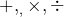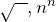Welcome to The Mathenæum Blog

Welcome to the The Mathenæum Blog — companion site to my mathematics learning and exploration website The Mathenæum.

Although I am not teaching at the moment, I have not lost my interest in mathematics education, or the strong desire …

In an earlier post, we looked at expanding binomial products. Remember how the result of the expansion is a mixture of the inputs — the numbers in the input do not appear in the result, but the numbers that …

How many tunes?

Beginning in 2008, there was quite a hubbub in the Australian music industry. The publishing company, Larrikin Music, sued the iconic Australian band Men At Work claiming that the flute riff for their hit song Down Under was taken from …

Probability is all about counting. The basic problem in probability is to work out how likely something is by counting how many ways it can happen, and then comparing that number to how many ways any ways anything can happen. …

Ratios — calculations from the dawn of mathematics

Ratios and fractions are very similar things, since they are both arise from comparing the relative sizes of quantities. Let’s quickly review what exactly a fraction is. If you think of a “whole” as made up of some number of …

Recurring decimals are cool!

I was thinking about maths teaching the other day (I’m sure you’ll not be surprised to know this is something I often do) and in particular about the different approaches teachers bring to the subject. Teaching maths is a challenge …

Puzzles with 4 numbers, and the amazing power of powers

A fun puzzle is to choose a number between 1 and 10, then using that number exactly four times in an expression involving basic mathematical operations, i.e. at leastbut also possibly, see what values you can create.

For …

Solving Equations

A typical maths textbook presents equation solving in a very ordered, structured way. Starting with one-step equations such as, they introduce the single step solution processbefore moving onto two-step equations such as. For these equations …

Expanding Binomial Products

Welcome to the first mathematical post in The Mathenæum Blog. In this post, I have chosen to discuss expanding binomial products, because in many ways binomial products act as a bridge between elementary algebraic work, and more advanced applications …